Self-similar Network Traffic

The observation that traffic in packet-switched networks shows self-similar and long-range dependent behaviour goes back to local area Ethernet investigations made by Willinger et al. . In the sequel, a lot of empiric studies have been made that confirm the self-similar nature of packet-switched traffic in local and wide area networks and for different kinds of applications . While exact or asymptotic self-similarity does not always exactly fit to observed empiric traffic samples, it still comes closer to empiric observations than Poisson-based traffic models do. In particular, the effects of burstiness at different time scales and long-range dependence (see below) cannot be modelled by Poisson traffic models.

Mathematically speaking, let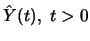be the number of packets traversing a link during the time interval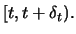Then the properties of the sequence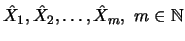with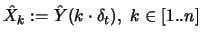are investigated.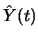and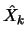are assumed to be realizations of stationary stochastic processes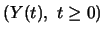and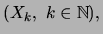respectively. In the following, the description of the properties of self-similar time series will concentrate on the theoretical valuesand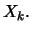It should be clear, that the empirically observed number of packets must be interpreted as realizations of these stochastic processes.

For the analysis of the properties of the time seriesit makes sense to introduce the scaled sample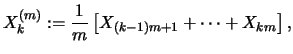the scaled variance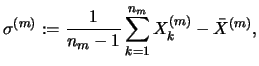where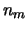denotes the number of scaled samples (it is assumed that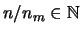) and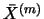denotes the mean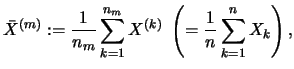and the autocorrelation functions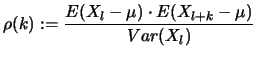and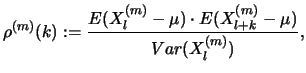respectively.

The following definition is taken from .
Definition: The time series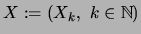is called asymptotically second order self-similar with self-similarity parameter or Hurst parameter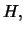if the second-order statistics of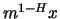converge as follows: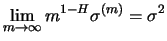for some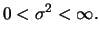(1)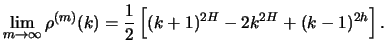(2)

If equities (1) and (2) are valid for all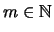and not only for the limit, the time series is said to be exactly self-similar of second order.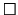For the modeling of traffic in packet-switched networks, only the range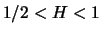is relevant. With the presented mathematical framework, the main properties of self-similar time series with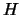within this range can be expressed as follows 

• burstiness at different time scales / high variability
The variability of the samples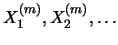remains high with increasing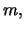which means that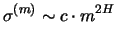for large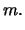• slowly decaying autocorrelation function
Slowly decaying in this context means that the autocorrelation function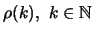of the stochastic processdecays as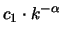for large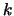with some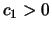and some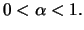In contrast, Poisson traffic has exponential decay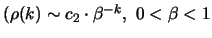for large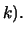As a consequence, for long-range-dependent traffic, we have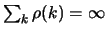(for Poisson traffic: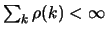).

There are a variety of methods for the generation of self-similar and long-range dependent traffic for simulation experiments:

1. Superposition of alternating renewal processes with power-tailed soujourn distributions
An alternating renewal process has two states ON and OFF which are alternately visited. The superposition of multiple ON-OFF-processes with power-tailed sojourn distributions leads to a good approximation of self-similar and long-range dependent traffic. The resulting stochastic processes are not exactly self-similar but asymptotically self-similar (see [6,2,8] for details).

2.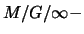queueing system based generation
If the service time distribution of anqueueing system has infinite variance, then the queue length process is long-range dependent and will yield a good approximation to a self-similar process .

3. Fractional Gaussian noise and fractional Brownian motion
Fractional Gaussian noise (FGN) and fractional Brownian motion (FBM) play an important role for experiments using the superposition of alternating renewal processes as self-similar traffic generators, since the limiting properties of the superposition method can be expressed in terms of FBM . For a survey on approaches for synthetic generation of FGN and a wavelet-based approach for FGN generation see .

4. Methods based on modulated Poisson processes
Two other approaches based on a distinct selection of the rate function of a modulated Poisson processe (also called doubly stochastic Poisson processes, DSPP) are shown in : The first approach uses the superposition of multiple fractal renewal processes as the rate function of a Poisson process (fractal-binomial-noise-driven Poisson process, FBNDP). The second approach which is called fractal-shot-noise-driven Poisson point process (FSNDP) uses a fractal shot noise process for the rate function of the Poisson process.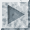Next: Bibliography